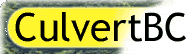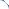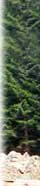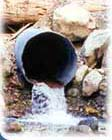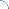### Home > Classroom > Notes on basic hydraulics

by Dr. S.O. Russell

There are two basic types of flow:

CLOSED CONDUIT or PIPE FLOW, where the water is under pressure and, provided the pressure is high enough, the pipes can run up or down hill; and

OPEN CHANNEL FLOW, where the water can only flow downhill. Open channel flow can be subdivided into:
uniform or non-uniform flow; and supercritical or subcritical.

Uniform flow almost never occurs in natural rivers, but since it is much easier to calculate uniform flow than non-uniform flow, we generally assume (for calculation purposes) that the flow is uniform. With uniform flow the most commonly used formula in North America is the Manning formula.

MANNING FORMULA

v = (R2/3S1/2)/n

where:
v is the average velocity,
S is the slope and n is the channel roughness (often called Manning’s n).

R =A/P
where:
A is the cross-section area and
P is the wetted perimeter, which is often approximated as the top width of the channel.

Q =Av, where Q is the flow in m3/s.

A, P and S can be obtained from field measurements and n can be obtained from hydraulics textbooks or guidebooks.

Critical flow is a special case, where the flow is a maximum for the "energy" in the water. The energy E = d +v2/2g, where d is the depth, v the velocity and g the gravity constant. V2/2g is often referred to as the velocity head.

Subcritical flow (the more common type of flow) occurs when the velocity is less than critical (and the channel is fairly flat); and supercritical flow occurs when the velocity is greater than the critical velocity, which means that the channel has to be relatively steep. For example culverts usually have supercritical flow if the slope is greater than about 1.5%. We can use this to compute flows from water levels upstream of steep culverts, which have what is known as "entrance control" and to compute the flow that such a culvert can carry.

Subcritical flow can only change to supercritical flow by going through critical flow; and supercritical flow can only change back to subcritical flow by going through a hydraulic jump. This has practical importance in that if the flow goes through critical flow, then the flow can be calculated knowing the upstream water level and the channel geometry at the critical section.

In natural streams, supercritical flow can only occur locally, such as between the pools in tumbling flow in steep creeks. Otherwise the velocity would built up to the point where it can move quite large boulders – and in effect steep streams move their beds around to increase the roughness and prevent supercritical flow from developing over any significant lengths. We can use this to estimate flows in steep creeks.

[ ^ top of page ]home | classroom | tools | case studies | publications | links | BC map data | feedback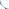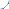stats